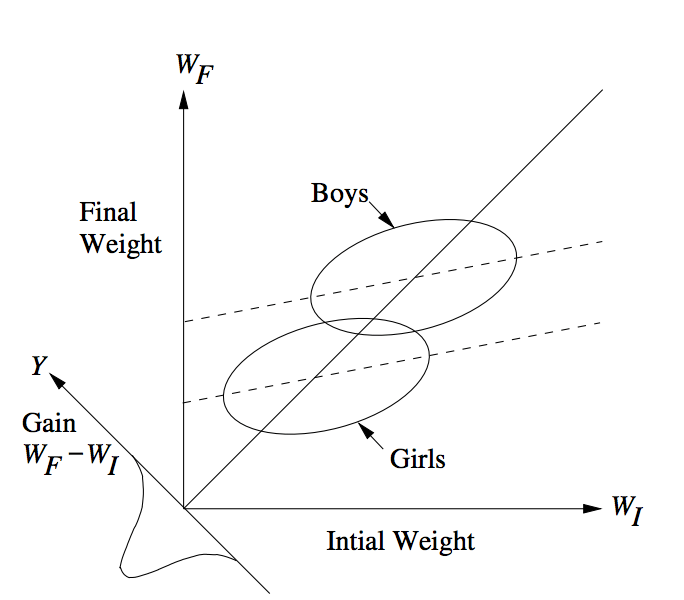Lord’s Paradox, which is a special instance of Simpson’s Paradox, is as follows (excerpted from Lord’s original paper):

“A large university is interested in investigating the effects on the students of
the diet provided in the university dining halls and any sex difference in these
effects. Various types of data are gathered. In particular, the weight of each
student at the time of his arrival in September and his weight the following June
are recorded

At the end of the school year, the data are independently examined by two
statisticians. Both statisticians divide the students according to sex. The first
statistician examines the mean weight of the girls at the beginning of the year and
at the end of the year and finds these to be identical. On further investigation,
he finds that the frequency distribution of weight for the girls at the end of the
year is actually the same as it was at the beginning.

He finds the same to be true for the boys. Although the weight of individual
boys and girls has usually changed during the course of the year, perhaps by a
considerable amount, the group of girls considered as a whole has not changed
in weight, nor has the group of boys. A sort of dynamic equilibrium has been
maintained during the year.The whole situation is shown by the solid lines in the diagram [Fig. 1]. Here
the two ellipses represent separate scatter-plots for the boys and the girls. The
frequency distributions of initial weight are indicated at the top of the diagram
and the identical distributions of final weight are indicated on the left side. People
falling on the solid 45 degree line through the origin are people whose initial and final
weight are identical. The fact that the center of each ellipse lies on this 45 degree
line represents the fact that there is no mean gain for either sex.

The first statistician concludes that as far as these data are concerned, there is
no evidence of any interesting effect of the school diet (or of anything else) on
student. In particular, there is no evidence of any differential effect on the two
sexes, since neither group shows any systematic change.

The second statistician, working independently, decides to do an analysis of covariance.
After some necessary preliminaries, he determines that the slope of
the regression line of final weight on initial weight is essentially the same for
the two sexes. This is fortunate since it makes possible a fruitful comparison of
the intercepts of the regression lines. (The two regression lines are shown in the
diagram as dotted lines. The figure is accurately drawn, so that these regression
lines have the appropriate mathematical relationships to the ellipses and to the
45 degree line through the origin.) He finds that the difference between the intercepts
is statistically highly significant.

The second statistician concludes, as is customary in such cases, that the boys
showed significantly more gain in weight than the girls when proper allowance
is made for differences in initial weight between the two sexes. When pressed to
explain the meaning of this conclusion in more precise terms, he points out the
following: If one selects on the basis of initial weight a subgroup of boys and a
subgroup of girls having identical frequency distributions of initial weight, the
relative position of the regression lines shows that the subgroup of boys is going
to gain substantially more during the year than the subgroup of girls.

The college dietician is having some difficulty reconciling the conclusions of the
two statisticians. The first statistician asserts that there is no evidence of any
trend or change during the year for either boys or girls, and consequently, a
fortiori, no evidence of a differential change between the sexes. The data clearly
support the first statistician since the distribution of weight has not changed for
either sex.

The second statistician insists that wherever boys and girls start with the same
initial weight, it is visually (as well as statistically) obvious from the scatter-plot
that the subgroup of boys gains more than the subgroup of girls.”

This paradox arises from the fact that using a regression line when there are uncontrolled preexisting differences between naturally occurring groups may or may not be appropriate. Basically, the boys weighed more than girls at the beginning and gained more weight. It could be that they gained more weight because they were boys or that they gained more weight because they weighed more to start with. It is not possible to tell which is which.

Some articles that discuss this are here (source for the graph) and here.